This is the rendered collections of notes by me, Lex Toumbourou.

You can find the source on the GitHub project.

The notes are collecting using my interpretation of the Zettelkasten method.

More
• ## Law of cosines (Khan Academy)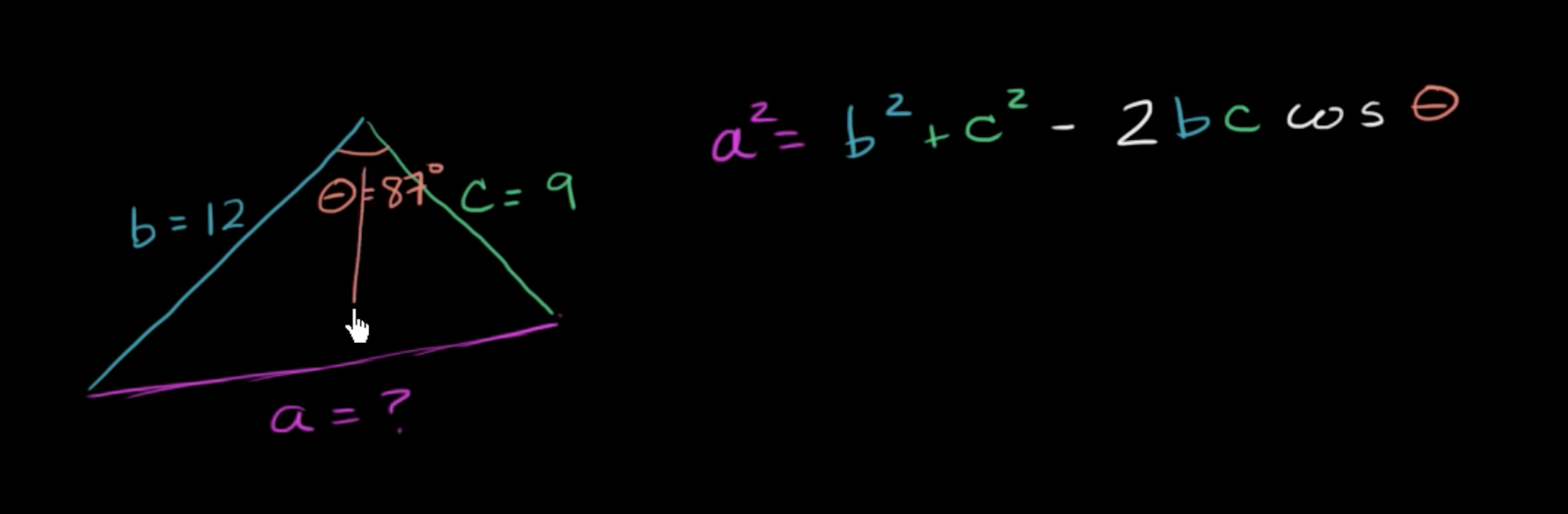Notes from the Khan Academy video series on the Law of Cosines

• ## Roblox Vector3

In Roblox, a Vector3 defines a Vector with three components. We use it for setting the position, rotation, and size of elements.

For example, a …

• ## Deep Learning for Coders (2020)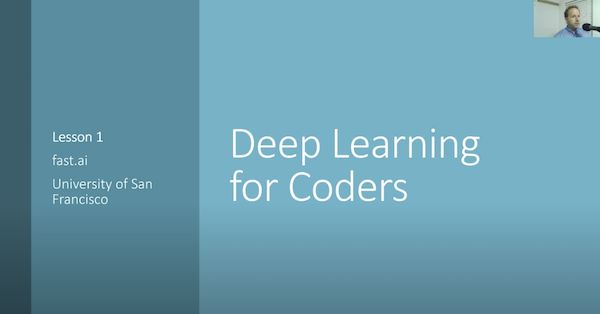Notes from the Deep Learning for Coders (2020) video series by Jeremy Howard and Sylvain Gugger (fast.ai)

• ## Matrix Multiplication

Matrix multiplication is a mathematical operation between 2 matrices that returns a matrix.

For each row in the first matrix, take the Dot Productf …

• ## Dot Product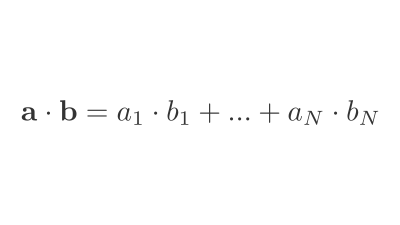An operation between 2 vectors that returns a number.

• ## Binary Cross-Entropy Loss

Binary Cross-Entropy (BCE), also known as log loss, is a loss function used in binary or multi-label machine learning training.

It's nearly identical to Negative …

• ## Presizing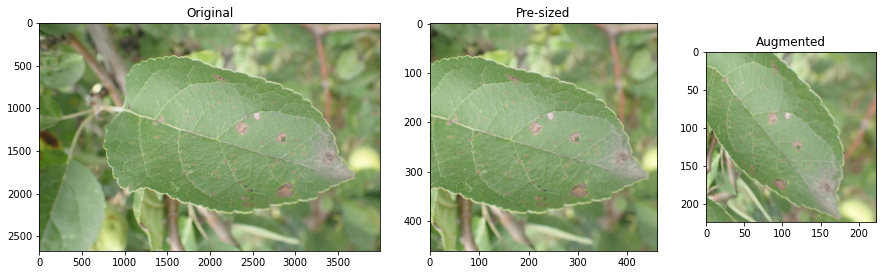Pre-sizing is a technique for preparing images for training a neural network.

In standard image pipelines, images are resized to a size suitable for our …

• ## Categorical Cross-Entropy Loss

Categorical Cross-Entropy Loss Function, also known as Softmax Loss, is a loss function used in multiclass classification model training. It applies the Softmax Activation Function …

• ## Cross-Entropy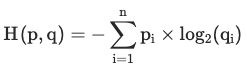Cross-entropy measures the average number of bits required to identify an event if you had a coding scheme optimised for one probability distribution $q …$

• ## Information EntropyEntropy is a measure of uncertainty of a random variable's possible outcomes.

It's highest when there are many equally likely outcomes. As you introduce more …